Download Presentation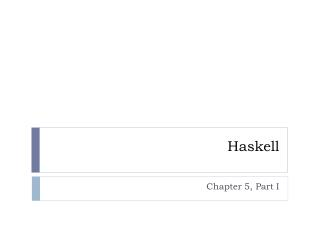# Haskell - PowerPoint PPT Presentation

Haskell. Chapter 5, Part I. Topics. Higher Order Functions map, filter Infinite lists. Higher Order Functions. A function that can take functions as parameters OR A function that returns a function as resultI am the owner, or an agent authorized to act on behalf of the owner, of the copyrighted work described.
Download PresentationAn Image/Link below is provided (as is) to download presentation

Download Policy: Content on the Website is provided to you AS IS for your information and personal use and may not be sold / licensed / shared on other websites without getting consent from its author.While downloading, if for some reason you are not able to download a presentation, the publisher may have deleted the file from their server.

- - - - - - - - - - - - - - - - - - - - - - - - - - E N D - - - - - - - - - - - - - - - - - - - - - - - - - -
Presentation Transcript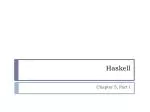Chapter 5, Part ITopics
• Higher Order Functions
• map, filter
• Infinite lists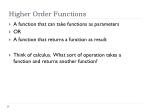Higher Order Functions
• A function that can take functions as parameters
• OR
• A function that returns a function as result
• Think of calculus. What sort of operation takes a function and returns another function?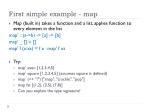First simple example - map
• Map (built in) takes a function and a list, applies function to every element in the list

map' :: (a->b) -> [a] -> [b]

map' _ [] = []

map' f (x:xs) = f x : map' f xs

• Try:
• map’ even [1,2,3,4,5]
• map’ square [1,2,3,4,5] (assumes square is defined)
• map’ (++ "!") ["snap", "crackle", "pop"]
• map fst [(1,2), (3,5), (7,8)]
• Can you explain the type signature?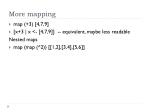More mapping
• map (+3) [4,7,9]
• [x+3 | x <- [4,7,9]] -- equivalent, maybe less readable

Nested maps

• map (map (^2)) [[1,2],[3,4],[5,6]]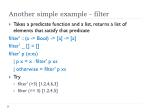Another simple example - filter
• Takes a predicate function and a list, returns a list of elements that satisfy that predicate

filter' :: (a -> Bool) -> [a] -> [a]

filter' _ [] = []

filter' p (x:xs)

| p x = x : filter' p xs

| otherwise = filter' p xs

• Try
• filter' (>3) [1,2,4,6,3]
• filter (== 3) [1,2,4,5]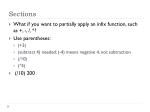Sections
• What if you want to partially apply an infix function, such as +, -, /, *?
• Use parentheses:
• (+3)
• (subtract 4) needed; (-4) means negative 4, not subtraction
• (/10)
• (*5)
• (/10) 200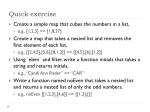Quick exercise
• Create a simple map that cubes the numbers in a list,
• e.g., [1,2,3] => [1,8,27]
• Create a map that takes a nested list and removes the first element of each list,
• e.g., [[2,4,5],[5,6],[8,1,2]] => [[4,5],,[1,2]]
• Using `elem` and filter, write a function initials that takes a string and returns initials,
• e.g., “Cyndi Ann Rader” => “CAR”
• Write a function named noEven that takes a nested list and returns a nested list of only the odd numbers.
• e.g., noEven [[1,2,3],[4,6]] => [[1,3],[]]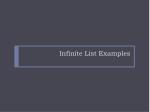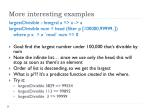More interesting examples

largestDivisible :: Integral a => a -> a

largestDivisible num = head (filter p [100000,99999..])

where p x = x `mod` num == 0

• Goal: find the largest number under 100,000 that’s divisible by num
• Note the infinite list… since we use only the head, this will stop as soon as there’s an element
• Order of list is descending, so we get the largest
• What is p??? It’s a predicate function created in the where.
• Try it:
• largestDivisible 3829 => 99554
• largestDivisible 113 => 99892
• largestDivisible 3 => 99999takeWhile
• takeWhile takes a predicate and a list, returns elements as long as the predicate is true
• sum (takeWhile (<10) [1..100])
• takeWhile (/= ' ') "what day is it?"
• Find the sum of all odd squares < 10,000
• need to create squares
• select only odd squares
• stop when square >= 10,000
• sum (takeWhile (<10000) (filter odd (map (^2) [1..])))
• OR
• sum (takeWhile (<10000) [m | m <- [n^2 | n <- [1..]], odd m])A fun problem
• Collatz sequence/chain
• If the number is 1, stop
• If the number is even, divide it by 2
• If the number is odd, multiply it by 3 and add 1
• Repeat with the result number
• Example: start with 13 -> 40 -> 20 -> 10 -> 5 -> 16 -> 8 -> 4 -> 2 -> 1
• Mathematicians theorize that for all starting numbers, the chain will finish at the number 1.
• Our goal: for all starting numbers between 1 and 100, how many have Collatz chains with length > 15?

Think about: what kinds of problems are we solving here?The code

chain :: Integer -> [Integer]

chain 1 = 

chain n

| even n = n : chain (n `div` 2)

| odd n = n : chain (n*3 + 1)

numLongChains :: Int

numLongChains = length (filter isLong (map chain [1..100]))

where isLongxs = length xs > 15Thunks*
• Lists are lazy
• [1,2,3,4] is really 1:2:3:4:[]
• When first element is evaluated (e.g., by printing it), the rest of the list 2:3:4:[] is a promise of a list – known as a thunk
• A thunk is a deferred computation

* from chapter 9Curried Functions
• Every function in Haskell officially takes one parameter
• So, how have we been able to do functions that take two parameters?
• They are curried functions.
• Always take exactly one parameter
• When called with that parameter, it returns a function that takes the next parameter
• etc. until all parameters usedA curried example
• :t max => max :: Ord a => a -> a -> a
• equivalent to max :: (Ord a) => a -> (a -> a)
• max 4 5 === (max 4) 5
• (max 4) returns a partially applied function
• Try: (max ((max 4) 5)) 3

max

max 4 _

4

max 5 _

max 4

5

max 5

3

5Can’t show a function

*Main> (max 4)

<interactive>:88:1:

No instance for (Show (a0 -> a0))

arising from a use of `print'

Possible fix: add an instance declaration for (Show (a0 -> a0))

In a stmt of an interactive GHCi command: print it

• What??
• (max 4) produced a function of type (a0 -> a0)
• But functions aren’t instances of Show, so GHCi doesn’t know how to display

Compare to Scheme:Another example

multThree :: Int -> Int -> Int -> Int

multThree x y z = x * y * z

• multThree 3 5 9 => 135
• ((multThree 3) 5) 9

* I’m not saying this is how it’s implemented… just a way to think about it…

multThree

multThree 3

3

multThree 3

5

multThree 15

multThree 15

9

135multThree continued

multThree :: Int -> Int -> Int -> Int

multThree x y z = x * y * z

• multThree 3 5 9 => 135
• ((multThree 3) 5) 9
• multThree :: Int -> (Int -> (Int -> Int)) – equivalent
• function takes Int, returns function of type (Int -> (Int ->Int))
• that function takes an Int, returns function of type (Int -> Int)
• that function takes an Int, returns an Int

multThree

multThree 3

3

A

multThree 3

5

multThree 15

B

multThree 15

C

9

135Take advantage of currying

You can store a partially applied function:

*Main> let multTwoWithNine = multThree 9

*Main> multTwoWithNine 2 3

54

multTwoWithNine

multThree

multThree 9 _ _

9

multTwoWithNine

multTwoWithNine

(9) 2 _

2

multTwoWithNine

9 2 _

54

3How could we use this?

tenPctDiscount

multThreeF

multThreeF 10 _ _

.10

tenPctDiscount

4

tenPctDiscount

4

2

5

What if you wanted a 20% discount? 25%?

Write the code in Play and ShareAnother Example

doubleArea

multThree

multThree 2

2

doubleArea

4

doubleArea 4

40

5

Write the code in Play and SharePlay and Share
• Write a function doubleArea that takes a width and height and returns 2 * the area – making use of multThree (could clearly be done directly, but use multThree for curry practice).
• doubleArea 4 5 => 40
• Write a function multThreeF that works with floating point values. (hint: use Num a as a class constraint)
• Write a function tenPctDiscount that takes two numbers and calculates a 10% discount (using your multThreeF, of course)
• tenPctDiscount 4 5 => 2.0
• Write a function named pctDiscount that takes a floating point and returns a partially applied function. Usage:
• *Main> let sale = pctDiscount 0.5
• *Main> sale 4 5
• 10.0
• *Main> (pctDiscount 0.5) 4 5
• 10.0More Play and Share
• Curried functions are convenient with map
• Write a function total that takes a discount % and two numbers stored in a list, and returns the discount amount
• total 0.1 [4,5] => 2.0
• Write a function totalTenPctthat returns a partially applied total function with discount set to 0.1
• Try using this with map:
• map totalTenPct [[4,5],[8,10]] => [2.0, 8.0]
• Play with other uses of map, e.g.,
• map (multThree 3 4) [5,6,7]